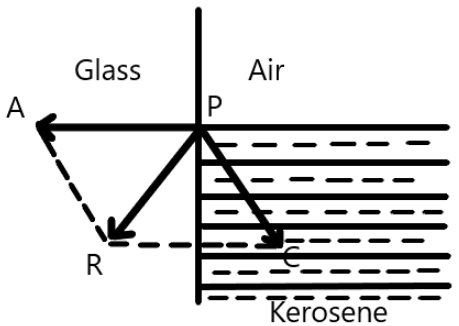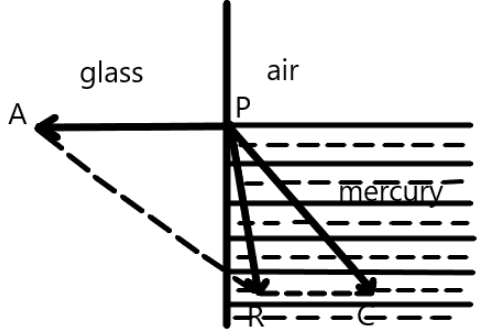Explain why the angle of contact is acute for kerosene-glass pair and obtuse for Mercury- glass .

Dear Student
Consider kerosene which is in contact with the glass.Here, P is the molecule that is deflected in the liquid.

Here, the adhesive force between the pair will be very large as compared to the cohesive force. Therefore, the resultant of the forces will be directed into the solid. Now, as we know, the free surface of a liquid that is at rest will be perpendicular to the resultant of the force on it. Therefore, the molecule P will move upward until the surface of the liquid will become perpendicular to the PR Therefore, the shape of the liquid will become concave and the tangent to the surface will become an acute angle with the surface.
Now, consider mercury which is in contact with the glass pair.Now, as we know that mercury is a non-wetting liquid, therefore, the cohesive force between the pair will be large as compared to the adhesive force. Therefore, the resultant of the forces will be towards liquid. The molecule P will move downwards until the surface of the liquid will become perpendicular to PR . Hence, the liquid will acquire a convex shape and the tangent to the surface will become an obtuse angle with the surface of the liquid.

Hence, the angle of the kerosene-glass pair is an acute angle and the angle of the mercury-glass pair is an obtuse angle.

Regards

• 1
What are you looking for?